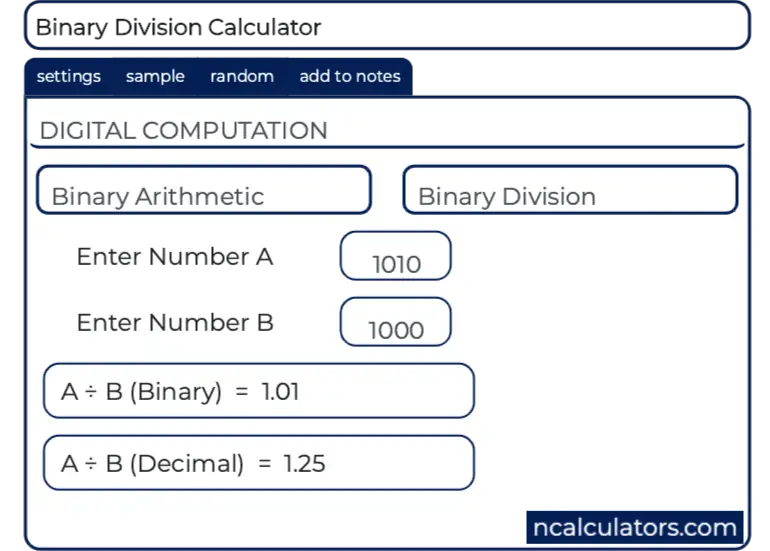# Online Binary Long Division Calculator## Galois Field GF(2) Calculator

Binary values representing polynomials in GF(2) can readily be manipulated using the rules of modulo 2 arithmetic on 1-bit coefficients.

This online tool serves as a polynomial calculator in GF(2). Be aware that this is not an ordinary binary calculator.

Enter binary values for two input operands and choose an operation.

## Dividing Binary Numbers

Select Polynomials to see the result in polynomial form. Select Discussion to see a detailed explanation of the underlying arithmetic.

1100101 --------- = 1001, Remainder = 0 1101

## Detailed Calculation

 1001 --------- 1101 ) 1100101 1101 ---- 001101 1101 ---- 0000

## MATLAB Example

Calculations in GF(2) are performed by MATLAB functions found within the Communications Toolbox.

>> a = gf( [1 1 0 0 1 0 1] );

>> b = gf( [1 1 0 1] );

>> [q,r] = deconv(a,b) % deconvolution is equivalent to division

q = GF(2) array.

Array elements =

1      0      0      1

r = GF(2) array.

Array elements =

0